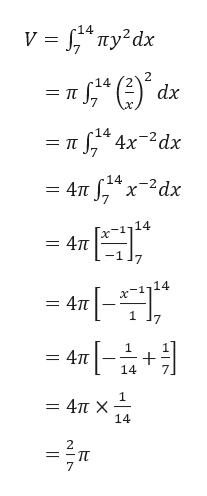# Consider the solid obtained by rotating the region bounded by the given curves about the x-axis.y = 2/x, x = 7, x = 14, y = 0 Find the volume V of this solid.

Question
23 views

Consider the solid obtained by rotating the region bounded by the given curves about the x-axis.
y = 2/x, x = 7, x = 14, y = 0
Find the volume V of this solid.

check_circle

Step 1

It is given that the region R is bounded by the curve y = 2/x and the lines x = 7, x = 14 and y = 0. This ...help_outlineImage Transcriptionclosev y?dx -14 V 14 dx 4x 2dx 14 4TTx2dx 14 4Tt x-1114 4Tt 7 1 4Tt 14 1 - 4π Χ 14 = -1 7 fullscreen

### Want to see the full answer?

See Solution

#### Want to see this answer and more?

Solutions are written by subject experts who are available 24/7. Questions are typically answered within 1 hour.*

See Solution
*Response times may vary by subject and question.
Tagged in

### Integration